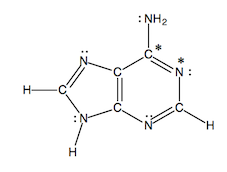Problem: How many sigma and how many pi bonds are present in adenine? 14 sigma and 4 pi 18 sigma and 0 pi 11 sigma and 4 pi 16 sigma and 4 pi 12 sigma and 2 pi

82% (60 ratings)
Problem Details

How many sigma and how many pi bonds are present in adenine?

1. 14 sigma and 4 pi
2. 18 sigma and 0 pi
3. 11 sigma and 4 pi
4. 16 sigma and 4 pi
5. 12 sigma and 2 piWhat scientific concept do you need to know in order to solve this problem?

Our tutors have indicated that to solve this problem you will need to apply the Lewis Dot Structures: Sigma & Pi Bonds concept. You can view video lessons to learn Lewis Dot Structures: Sigma & Pi Bonds Or if you need more Lewis Dot Structures: Sigma & Pi Bonds practice, you can also practice Lewis Dot Structures: Sigma & Pi Bonds practice problems .

What is the difficulty of this problem?

Our tutors rated the difficulty of How many sigma and how many pi bonds are present in adenine?... as medium difficulty.

How long does this problem take to solve?

Our expert Chemistry tutor, Sabrina took 3 minutes to solve this problem. You can follow their steps in the video explanation above.

What professor is this problem relevant for?

Based on our data, we think this problem is relevant for Professor Showalter's class at PSU.